# Quantitative Aptitude Quiz for SSC(Simple Interest): 2 April 2021

Updated Fri, 02 Apr 2021 05:21 PM ISTSource: safalta.com
1. A person borrows Rs. 5000 for 2 years at 4% p.a. simple interest. He immediately lends it to another person at 6 (1/4)% p.a for 2 years. Find his gain in the transaction per year

### Free Demo Classes

a) Rs. 112.50
b) Rs. 125
c) Rs. 167.50
d) Rs. 225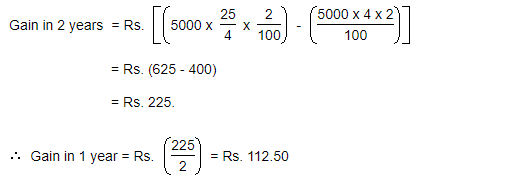2. A certain amount earns simple interest of Rs. 1750 after 7 years. Had the interest been 2% more, how much more interest would it have earned?

a) Rs. 35
b) Rs. 140
c) Rs. 70
d) Cannot be determined

We need to know the S.I., principal and time to find the rate.

Since the principal is not given, so data is inadequate.

3. What will be the ratio of simple interest earned by certain amount at the same rate of interest for 6 years and that for 9 years?

a) 3 : 2
b) 2 : 3
c) 3 : 4
d) 4 : 3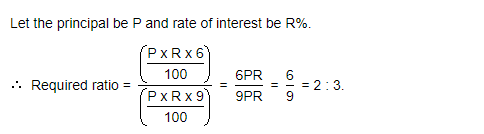4. A sum of money amounts to Rs. 9800 after 5 years and Rs. 12005 after 8 years at the same rate of simple interest. The rate of interest per annum is:

a) 8%
b) 18%
c) 16%
d) 12%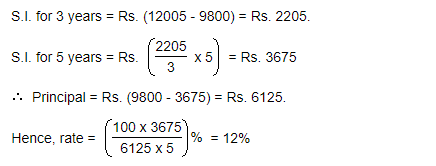5. A man took loan from a bank at the rate of 12% p.a. simple interest. After 3 years he had to pay Rs. 5400 interest only for the period. The principal amount borrowed by him was

a) Rs. 15,000
b) Rs. 24,000
c) Rs. 12,000
d) Rs. 18,000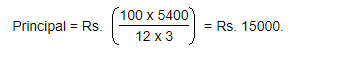6. A sum of Rs. 725 is lent in the beginning of a year at a certain rate of interest. After 8 months, a sum of Rs. 362.50 more is lent but at the rate twice the former. At the end of the year, Rs. 33.50 is earned as interest from both the loans. What was the original rate of interest?

a) 3.06%
b) 3.96%
c) 3.16%
d) 3.46%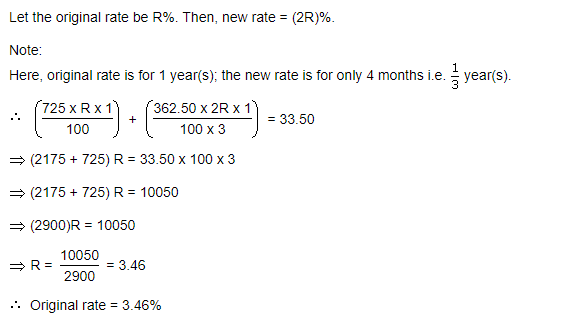7. A lent Rs. 5000 to B for 2 years and Rs. 3000 to C for 4 years on simple interest at the same rate of interest and received Rs. 2200 in all from both of them as interest. The rate of interest per annum is:

a) 18%
b) 16%
c) 12%
d) 10%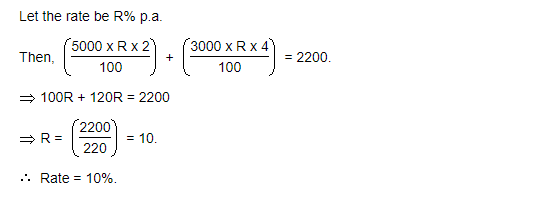8. An automobile financier claims to be lending money at simple interest, but he includes the interest every six months for calculating the principal. If he is charging an interest of 10%, the effective rate of interest becomes:

a) 10%
b) 10.5%
c) 10.25%
d) 10.75%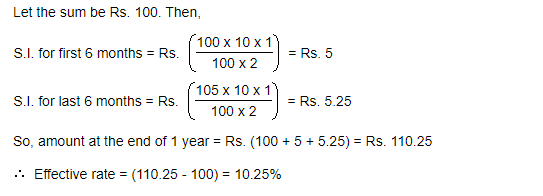9. A sum of Rs. 12,500 amounts to Rs. 15,500 in 4 years at the rate of simple interest. What is the rate of interest?

a) 4%
b) 6%
c) 8%
d) 10%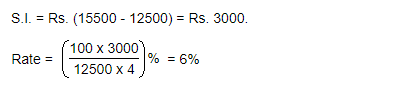10. Reena took a loan of Rs. 1200 with simple interest for as many years as the rate of interest. If she paid Rs. 432 as interest at the end of the loan period, what was the rate of interest?

a) 6%
b) 8%
c) 4%
d) 10%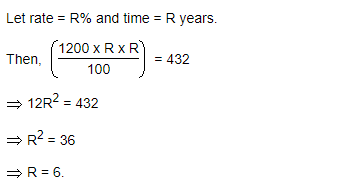### Disclaimer

अपनी वेबसाइट पर हम डाटा संग्रह टूल्स, जैसे की कुकीज के माध्यम से आपकी जानकारी एकत्र करते हैं ताकि आपको बेहतर अनुभव प्रदान कर सकें, वेबसाइट के ट्रैफिक का विश्लेषण कर सकें, कॉन्टेंट व्यक्तिगत तरीके से पेश कर सकें और हमारे पार्टनर्स, जैसे की Google, और सोशल मीडिया साइट्स, जैसे की Facebook, के साथ लक्षित विज्ञापन पेश करने के लिए उपयोग कर सकें। साथ ही, अगर आप साइन-अप करते हैं, तो हम आपका ईमेल पता, फोन नंबर और अन्य विवरण पूरी तरह सुरक्षित तरीके से स्टोर करते हैं। आप कुकीज नीति पृष्ठ से अपनी कुकीज हटा सकते है और रजिस्टर्ड यूजर अपने प्रोफाइल पेज से अपना व्यक्तिगत डाटा हटा या एक्सपोर्ट कर सकते हैं। हमारी Cookies Policy, Privacy Policy और Terms & Conditions के बारे में पढ़ें और अपनी सहमति देने के लिए Agree पर क्लिक करें।

Agree

Get free counselling session